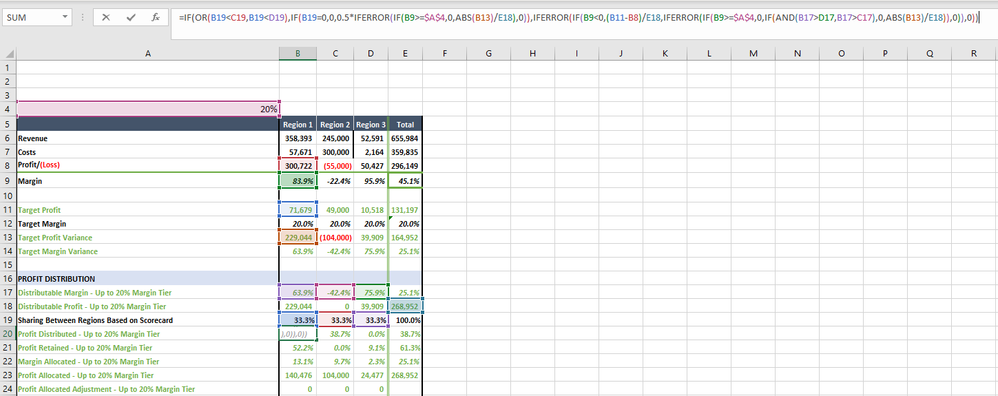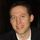cancel
Showing results for
Did you mean:

Fabric is Generally Available. Browse Fabric Presentations. Work towards your Fabric certification with the Cloud Skills Challenge.Resolver I

## Converting Lengthy, Complex Excel Formulas into DAX for Power BI Reporting Best Practices?

Hi all - I've been tasked with creating a data model in DAX/Power BI that replicates calculations from a complex Excel workbook which is full of conditionals, locked and relative cell references, column and row aggregations and other kinds of typical Excel formulas. Much of these are done to render a clean reporting view of the data, so I figured there's got to be a simpler, easier way to break down these calculations in DAX?

I've been able to make some progress on about half of it, but am stuck on the formula below.

I was curious to know if someone could offer some more generalized advice on how to approach these kinds of conversions or re-creations of Excel formulas. In Excel, I find many of the nested conditionals to be unreadable and confusing.

I'm somewhat adept at DAX, but certain concepts still present challenges for me, so I was hoping this would be a great learning opportunity to really improve my skills and knowledge.

Does anyone have any input or ideas about how best to approach solving for these kinds of conversion tasks from Excel into DAX?1 ACCEPTED SOLUTIONSuper User

I would start by creating a set of base measures such as Revenue, Costs, etc. Then use these base measures as building blocks for more complex measures. It appears that rows correlate to measures and columns slice by region. Cell B19 is referenced three times in the formula, so you could create a measure that is specific to Region 1 (use CALCULATE with a base measure, filtered for Region 1) and then use that measure in the final measure (cell B20).

The 20% factor can be specified via a parameter (Numeric range, formerly What-if).

If you want the ability to create custom rows that are calculations of other rows, as well as apply custom formatting, try the matrix visuals below:

Proud to be a Super User!

4 REPLIES 4Super User

Here are some tips that may help.

Use the SWITCH function instead of nested IFs.

Use variables for readability, reusability, and troubleshooting. When you create a variable, it can be used throughout the measure/calculated column. Variables are also helpful when troubleshooting. You can easily return a specific variable, allowing you to step through the code and find the error.

Proud to be a Super User!Resolver I

Thank you, the Excel JS formatter is fantastic; and I've been using the DAX Formatter for a while now.

What's the best practice to attempt to replicate this logic in DAX? Obviously, there are simple best practices like utilizing SWITCH and some more standardized operators, but to attempt to rebuild and redevelop this excel formula logic in DAX seems quite daunting, and I honestly have no idea where to even start?Super User

I would start by creating a set of base measures such as Revenue, Costs, etc. Then use these base measures as building blocks for more complex measures. It appears that rows correlate to measures and columns slice by region. Cell B19 is referenced three times in the formula, so you could create a measure that is specific to Region 1 (use CALCULATE with a base measure, filtered for Region 1) and then use that measure in the final measure (cell B20).

The 20% factor can be specified via a parameter (Numeric range, formerly What-if).

If you want the ability to create custom rows that are calculations of other rows, as well as apply custom formatting, try the matrix visuals below:

Proud to be a Super User!Resolver I

I've already done all of these things, and more with varying degrees of success. I think there's some improvements I can build into my DAX to ensure the ROW/FILTER Context for many of these measure calculations are correct.

It should be 73.7% under APAC and 73.7% under GLOBAL and instead, all three regions are zeroed out:For example, the following code I wrote calculates the correct percentage, but it doesn't attribute it to the correct region designation, only in the total of the regions combined.

Any ideas?

``````Profit Distributed - Up to 20% Margin Tier =
--- ### REGIONAL SCORECARD CALCULATION ### ---
VAR AMER_ScoreShare =
CALCULATE (
[Sharing Between Regions Based on Scorecard],
KPIs[Region] = "AMER"
)
VAR APAC_ScoreShare =
CALCULATE (
[Sharing Between Regions Based on Scorecard],
KPIs[Region] = "APAC"
)
VAR EMEA_ScoreShare =
CALCULATE (
[Sharing Between Regions Based on Scorecard],
KPIs[Region] = "EMEA"
) --- ### MARGIN ### ---
VAR AMER_Margin =
CALCULATE (
[Margin],
KPIs[Region] = "AMER"
)
VAR APAC_Margin =
CALCULATE (
[Margin],
KPIs[Region] = "APAC"
)
VAR EMEA_Margin =
CALCULATE (
[Margin],
KPIs[Region] = "EMEA"
) --- ### TARGET PROFIT VARIANCE ### ---
VAR AMER_TargetProfitVariance =
CALCULATE (
[Target Profit Variance],
KPIs[Region] = "AMER"
)
VAR APAC_TargetProfitVariance =
CALCULATE (
[Target Profit Variance],
KPIs[Region] = "APAC"
)
VAR EMEA_TargetProfitVariance =
CALCULATE (
[Target Profit Variance],
KPIs[Region] = "EMEA"
) --- ### TARGET PROFIT ### ---
VAR AMER_TargetProfit =
CALCULATE (
[Target Profit],
KPIs[Region] = "AMER"
)
VAR APAC_TargetProfit =
CALCULATE (
[Target Profit],
KPIs[Region] = "APAC"
)
VAR EMEA_TargetProfit =
CALCULATE (
[Target Profit],
KPIs[Region] = "EMEA"
) --- ### PROFIT LOSS ### ---
VAR AMER_ProfitLoss =
CALCULATE (
[Profit/(Loss)],
KPIs[Region] = "AMER"
)
VAR APAC_ProfitLoss =
CALCULATE (
[Profit/(Loss)],
KPIs[Region] = "APAC"
)
VAR EMEA_ProfitLoss =
CALCULATE (
[Profit/(Loss)],
KPIs[Region] = "EMEA"
) --- ### DISTRIBUTABLE MARGIN ### ---
VAR AMER_DistMarg =
CALCULATE (
[Distributable Margin - Up to 20% Margin Tier],
KPIs[Region] = "AMER"
)
VAR APAC_DistMarg =
CALCULATE (
[Distributable Margin - Up to 20% Margin Tier],
KPIs[Region] = "APAC"
)
VAR EMEA_DistMarg =
CALCULATE (
[Distributable Margin - Up to 20% Margin Tier],
KPIs[Region] = "EMEA"
)
VAR GLOBAL_DistProfit =
CALCULATE (
[Distributable Profit - Up to 20% Margin Tier],
KPIs[Region]
IN {
"AMER",
"APAC",
"EMEA"
}
) --- ### MARGIN CALCULATION BY REGION ### ---
VAR AMER_ProfitDistributed =
IF (
OR (
AMER_ScoreShare < APAC_ScoreShare,
AMER_ScoreShare < EMEA_ScoreShare
),
IF (
APAC_ScoreShare = 0,
0,
0.5
* IFERROR (
IF (
AMER_Margin >= [Margin Measure],
0,
ABS ( AMER_TargetProfitVariance )
/ CALCULATE (
[Distributable Profit - Up to 20% Margin Tier],
KPIs[Region]
IN {
"AMER",
"APAC",
"EMEA"
}
)
),
0
)
),
IFERROR (
IF (
AMER_Margin < 0,
( AMER_TargetProfit - AMER_ProfitLoss )
/ CALCULATE (
[Distributable Profit - Up to 20% Margin Tier],
KPIs[Region]
IN {
"AMER",
"APAC",
"EMEA"
}
),
IFERROR (
IF (
AMER_Margin >= [Margin Measure],
0,
IF (
AND (
AMER_DistMarg > EMEA_DistMarg,
AMER_DistMarg > APAC_DistMarg
),
0,
ABS ( AMER_TargetProfitVariance )
/ CALCULATE (
[Distributable Profit - Up to 20% Margin Tier],
KPIs[Region]
IN {
"AMER",
"APAC",
"EMEA"
}
)
)
),
0
)
),
0
)
)
VAR APAC_ProfitDistributed =
IF (
OR (
APAC_ScoreShare < AMER_ScoreShare,
APAC_ScoreShare < EMEA_ScoreShare
),
IF (
APAC_ScoreShare = 0,
0,
0.5
* IFERROR (
IF (
APAC_Margin >= [Margin Measure],
0,
ABS ( APAC_TargetProfitVariance )
/ CALCULATE (
[Distributable Profit - Up to 20% Margin Tier],
KPIs[Region]
IN {
"AMER",
"APAC",
"EMEA"
}
)
),
0
)
),
IFERROR (
IF (
APAC_Margin < 0,
( APAC_TargetProfit - APAC_ProfitLoss )
/ CALCULATE (
[Distributable Profit - Up to 20% Margin Tier],
KPIs[Region]
IN {
"AMER",
"APAC",
"EMEA"
}
),
IFERROR (
IF (
APAC_Margin >= [Margin Measure],
0,
IF (
AND (
APAC_DistMarg > AMER_DistMarg,
APAC_DistMarg > EMEA_DistMarg
),
0,
ABS ( APAC_TargetProfitVariance )
/ CALCULATE (
[Distributable Profit - Up to 20% Margin Tier],
KPIs[Region]
IN {
"AMER",
"APAC",
"EMEA"
}
)
)
),
0
)
),
0
)
)
VAR EMEA_ProfitDistributed =
IF (
OR (
EMEA_ScoreShare < APAC_ScoreShare,
EMEA_ScoreShare < AMER_ScoreShare
),
IF (
EMEA_ScoreShare = 0,
0,
0.5
* IFERROR (
IF (
EMEA_Margin >= [Margin Measure],
0,
ABS ( EMEA_TargetProfitVariance )
/ CALCULATE (
[Distributable Profit - Up to 20% Margin Tier],
KPIs[Region]
IN {
"AMER",
"APAC",
"EMEA"
}
)
),
0
)
),
IFERROR (
IF (
EMEA_Margin < 0,
( EMEA_TargetProfit - EMEA_ProfitLoss )
/ CALCULATE (
[Distributable Profit - Up to 20% Margin Tier],
KPIs[Region]
IN {
"AMER",
"APAC",
"EMEA"
}
),
IFERROR (
IF (
EMEA_Margin >= [Margin Measure],
0,
IF (
AND (
EMEA_DistMarg > AMER_DistMarg,
EMEA_DistMarg > APAC_DistMarg
),
0,
ABS ( EMEA_TargetProfitVariance )
/ CALCULATE (
[Distributable Profit - Up to 20% Margin Tier],
KPIs[Region]
IN {
"AMER",
"APAC",
"EMEA"
}
)
)
),
0
)
),
0
)
)
RETURN
AMER_ProfitDistributed + APAC_ProfitDistributed + EMEA_ProfitDistributed``````Announcements#### Power BI Monthly Update - November 2023

Check out the November 2023 Power BI update to learn about new features.#### Fabric Community News unified experience

Read the latest Fabric Community announcements, including updates on Power BI, Synapse, Data Factory and Data Activator.#### Exclusive opportunity for Women!

Join us for a free, hands-on Microsoft workshop led by women trainers for women where you will learn how to build a Dashboard in a Day!#### The largest Power BI and Fabric virtual conference

130+ sessions, 130+ speakers, Product managers, MVPs, and experts. All about Power BI and Fabric. Attend online or watch the recordings.Top Solution Authors
Top Kudoed Authors
Users online (3,693)